Courses

# Single Correct MCQ Of Solid State & Surface Chemistry, Past Year Questions JEE Advance, Class 12, Chemistry

## 14 Questions MCQ Test Class 12 Chemistry 35 Years JEE Mains &Advance Past yr Paper | Single Correct MCQ Of Solid State & Surface Chemistry, Past Year Questions JEE Advance, Class 12, Chemistry

Description
This mock test of Single Correct MCQ Of Solid State & Surface Chemistry, Past Year Questions JEE Advance, Class 12, Chemistry for JEE helps you for every JEE entrance exam. This contains 14 Multiple Choice Questions for JEE Single Correct MCQ Of Solid State & Surface Chemistry, Past Year Questions JEE Advance, Class 12, Chemistry (mcq) to study with solutions a complete question bank. The solved questions answers in this Single Correct MCQ Of Solid State & Surface Chemistry, Past Year Questions JEE Advance, Class 12, Chemistry quiz give you a good mix of easy questions and tough questions. JEE students definitely take this Single Correct MCQ Of Solid State & Surface Chemistry, Past Year Questions JEE Advance, Class 12, Chemistry exercise for a better result in the exam. You can find other Single Correct MCQ Of Solid State & Surface Chemistry, Past Year Questions JEE Advance, Class 12, Chemistry extra questions, long questions & short questions for JEE on EduRev as well by searching above.
QUESTION: 1

### CsBr has bcc structure with edge length 4.3. The shortestinter ionic distance in between Cs+ and Br– is : (1995S)

Solution:

For bcc structure, atomic radius,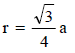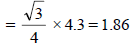Since, r = half the distance between two nearest
neighbouring atoms.
∴ Shortest inter ionic distance = 2 × 1.86 = 3.72

QUESTION: 2

### The coordination number of a metal crystallizing in ahexagonal close-packed structure is (1999 - 2 Marks)

Solution:

In a hcp structure atoms are located at the corners and at centres of two hexagons placed parallel to each other ; three more atoms are placed in a parallel plane midway between these two planes. Here each atom is surrounded by 12 others and is said to have
co-ordination number of 12.

QUESTION: 3

### In a solid ‘AB’ having the NaCl structure, ‘A’ atoms occupythe corners of the cubic unit cell. If all the face-centeredatoms along one of the axes are removed, then the resultantstoichiometry of the solid is     (2001S)

Solution:

(d) Effective number of ‘A’ atoms =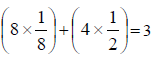Effective number of ‘B’ atoms =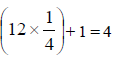∴ Formula of the solid = A3B4.

QUESTION: 4

A substance AxBy crystallizes in a face centred cubic (FCC)lattice in which atoms 'A' occupy each corner of the cubeand atoms 'B' occupy the centres of each face of the cube.Identify the correct composition of the substance AxBy   (2002S)

Solution:

Effective number of atoms at corners,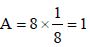Effective number of atoms at centre,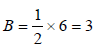Thus, the composition will be AB3

QUESTION: 5

Rate of physiorption increases with (2003S)

Solution:

Rate of physiorsption increases with decrease of temperature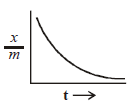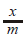= amt of gas adsorbed per unit

t = temperature

QUESTION: 6

Adsorption of gases on solid surface is generally exothermicbecause (2004S)

Solution:

When a gas is adsorbed on the surface, the freedom of movement of its molecules becomes restricted. This causes decrease in the entropy of the gas after adsorbtion, i.e. ΔS becomes negative

QUESTION: 7

In which of the following crystals alternate tetrahedral voidsare occupied? (2005S)

Solution:

In ZnS structure, sulphide ions occupy all FCC lattice points while Zn2+ ions are present in alternate tetrahedral voids.

QUESTION: 8

Lyophilic sols are (2005S)

Solution:

Lyophilic sols are self stabilizing because these sols are reversible and are highly hydrated in the solution.

QUESTION: 9

Among the following, the surfactant that will form micellesin aqueous solution at the lowest molar concentration atambient condition is : – (2008S)

Solution:

We know that surface acting agents (i.e. surfactants) such as soaps and detergents belong to the class of micelles. A miceller system when dissolved in water, dissociates to give ions. The anion consists of two parts. The polar groups such as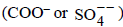ion
is water loving (i.e. hydrophilic) in nature. It is called head of the species. The hydrocarbon chain which is quite big in size is water repelling (i.e. hydrophobic) in nature. It is called tail of the species. The hydrocarbon
chain aggregates into the micelle above the critical concentration.
NOTE : It may also be noted that the critical
concentration for micelle formation decreases with increase in the molecular weight of the hydrocarbon chain of surfactant.
Here the two anions that are formed are in case of "B"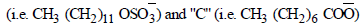The molecular weight of hydrocarbon chain is more in case of "B" so it has lower value of critical concentration for micelle formation in aqueous solution.
Hence the correct answer is option (b).

QUESTION: 10

Among the electrolytes Na2SO4, CaCl2, Al2(SO4)3 and NH4Cl, the most effective coagulating agent for Sb2S3 solis (2009S)

Solution:

As Sb2S3 is a negative sol, so Al2(SO4)3 will be the most effective coagulant due to higher positive charge on Al (Al3+) – Hardy-Schulze rule.

QUESTION: 11

The packing efficiency of the two-dimensional square unit cell shown below is :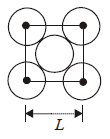Solution: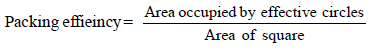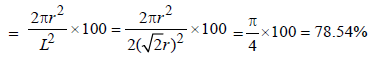QUESTION: 12

A compound MpXq has cubic close packing (ccp) arrangement of X. Its unit cell structure is shown below.
The empirical formula of the compound is      (2012 - I)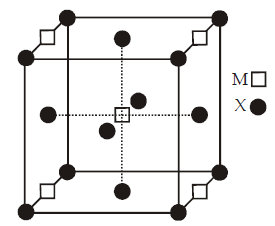Solution:

No. of M atoms =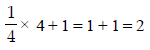No. of X atoms =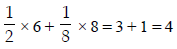So, formula = M2X4 = MX2

QUESTION: 13

The arrangement of X ions around A+ ion in solid AX is given in the figure (not drawn to scale). If the radius of X is 250 pm, the radius of A+ is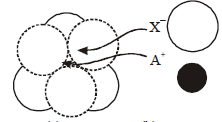Solution:

The arrangement given shows octahedral void
arrangement-limiting radius ratio for octahedral void is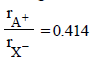QUESTION: 14

Methylene blue, from its aqueous solution, is adsorbed onactivated charcoal at 25°C. For this process, the correctstatement is (JEE Adv. 2013)

Solution:

The adsorption of methylene blue on activated charcoal is an example of physiosorption which is exothermic, multilayer and does not have energy barrier.